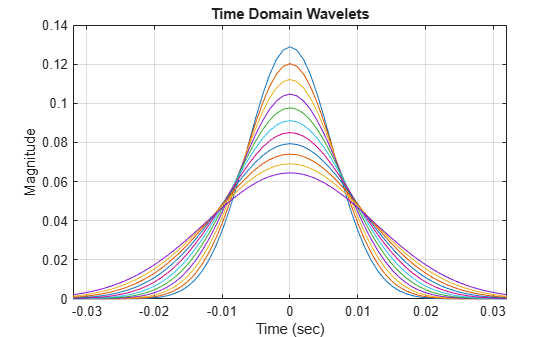# waveletsupport

CWT filter bank time supports

## Syntax

``spsi = waveletsupport(fb)``
``spsi = waveletsupport(fb,thresh)``

## Description

example

````spsi = waveletsupport(fb)` returns the wavelet time supports, defined as the time interval in which all of the wavelet's energy occurs. The default tolerance is 99.99% of the energy. The time supports are returned in the MATLAB® table `spsi`. The wavelets are normalized to have unit energy.```
````spsi = waveletsupport(fb,thresh)` specifies the threshold for the integrated energy. The time support of the wavelet is defined as the first instant the integrated energy exceeds `thresh` and the last instant the integrated energy is less than 1−`thresh`. If unspecified, `thresh` defaults to 10−4.```

## Examples

collapse all

Create a continuous wavelet transform filter bank. Set the sampling frequency to 1000 Hz and the frequency limits to range from 100 Hz to 200 Hz. Obtain the time supports of the wavelets in the filter bank.

```fb = cwtfilterbank('SamplingFrequency',1000,'FrequencyLimits',[100 200]); spsi = waveletsupport(fb)```
```spsi=11×5 table CF IsAnalytic TimeSupport Begin End ______ __________ ___________ ______ _____ 200 "Analytic" 0.032 -0.016 0.016 186.61 "Analytic" 0.034 -0.017 0.017 174.11 "Analytic" 0.038 -0.019 0.019 162.45 "Analytic" 0.04 -0.02 0.02 151.57 "Analytic" 0.042 -0.021 0.021 141.42 "Analytic" 0.046 -0.023 0.023 131.95 "Analytic" 0.048 -0.024 0.024 123.11 "Analytic" 0.052 -0.026 0.026 114.87 "Analytic" 0.056 -0.028 0.028 107.18 "Analytic" 0.06 -0.03 0.03 100 "Analytic" 0.064 -0.032 0.032 ```

Obtain the time domain wavelets from the filter bank and plot their magnitudes. Use the table to set the minimum and maximum limits of the x-axis to the smallest `Begin` value and largest `End` value, respectively.

```[psi,t] = wavelets(fb); plot(t,abs(psi)) grid on xlim([spsi.Begin(end) spsi.End(end)]) xlabel('Time (sec)') ylabel('Magnitude') title('Time Domain Wavelets')```## Input Arguments

collapse all

Continuous wavelet transform (CWT) filter bank, specified as a `cwtfilterbank` object.

Time support threshold for the wavelet, specified as a positive real number between 0 and 0.05. The time support of the wavelet is defined as the first instant the integrated energy of the wavelet exceeds `thresh` and the last instant the integrated energy is less than 1−`thresh`.

Data Types: `double`

## Output Arguments

collapse all

Wavelet time supports, returned as an Ns-by-`5` MATLAB table, where Ns is the number of wavelet bandpass frequencies (equal to the number of scales). The table has five variables:

Wavelet center frequency, returned as a positive real number.

Data Types: `double`

Wavelet designation, returned as a string. Wavelets that do not decay to 5% of their peak value at the Nyquist frequency are not considered analytic. The time support information for those wavelets are returned as `NaN`s.

Data Types: `string`

Wavelet time support, returned in samples, seconds, or MATLAB durations. The units of `TimeSupport` depend on whether you specify `SamplingFrequency` or `SamplingPeriod`. If you specify a `SamplingFrequency`, the units are seconds. If you specify a `SamplingPeriod`, the units are the same as the `SamplingPeriod`. If no `SamplingFrequency` or `SamplingPeriod` is specified, the units are samples.

Data Types: `double`

Beginning of wavelet time support, returned as an integer. `Begin` is defined as the first instant the wavelet integrated energy exceeds the default threshold, 10−4. `Begin` has the same units as `TimeSupport`.

Data Types: `double`

End of wavelet time support, returned as an integer. `End` is defined as the last instant the wavelet integrated energy is less than 1 − 10−4. `End` has the same units as `TimeSupport`.

Data Types: `double`

Data Types: `table`